# Expression Calculator

## Introduction

Version 2.0a 64-bit (10/2020)

With the calculator you can:

• Calculate expressions of an infinite length and complexity.
• Store an infinite number of lines containing expressions for reference or later use.
• Create an infinite number of user variables.
• Create an infinite number of user functions with up to three parameters.
• Save all the preceding possibilities for later use.
• Draw two-dimensional graphics.

Infinite means as long as your memory and the system can support it.

The main window is kept small. It can be moved over another program to see the values in it. There is a second window mode with more options. The window has a zoom function to make it bigger/smaller. It can draw and save a graph of functions.

## Screenshots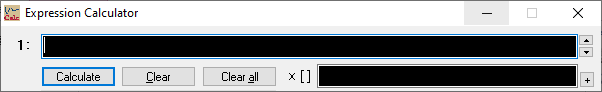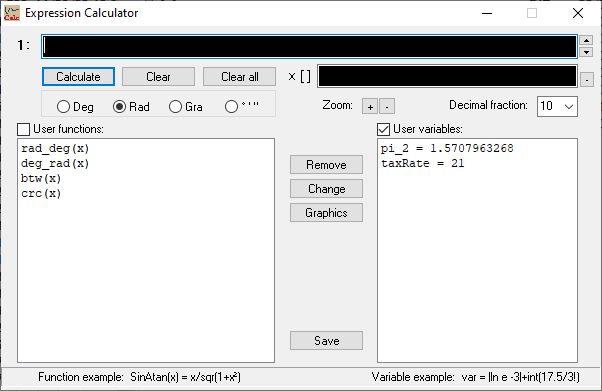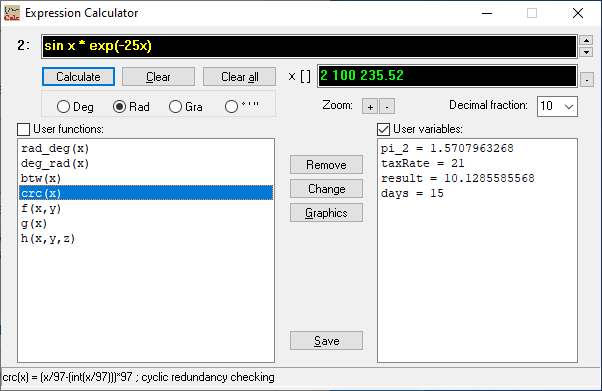## Graphics

• f(x) = tan x
• g(x) = sin x * exp(-.25x)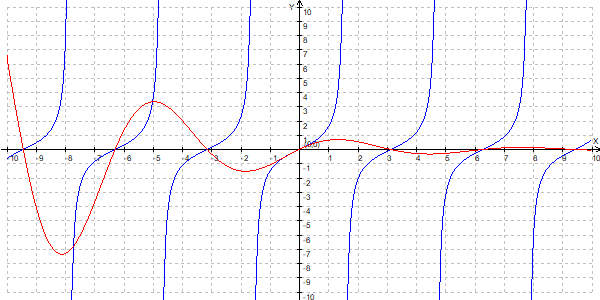• f(x) = (x²+1)/(2x³-4x-1)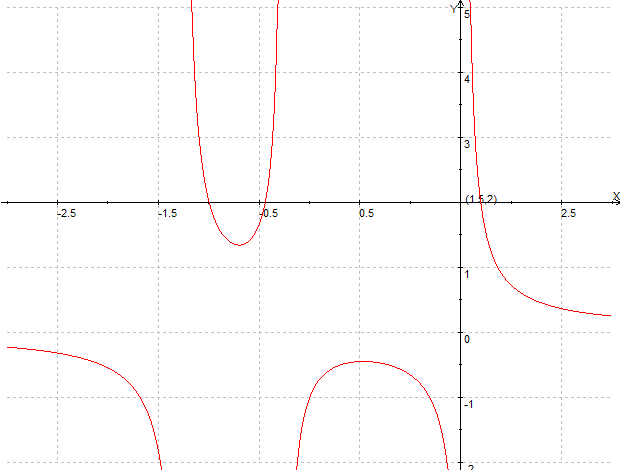## Examples

1 + 1
3 * sqr( 12001 + 12 / 5 )
23x² + 15x
sin( 2 pi / 15 )
7 + | -45 * 2.3 |
7 + abs( -45 * 2.3 )
12°3' + 15'34"
rad_deg( x ) = x * 180 / pi
f( x, y ) = x * 3 + y